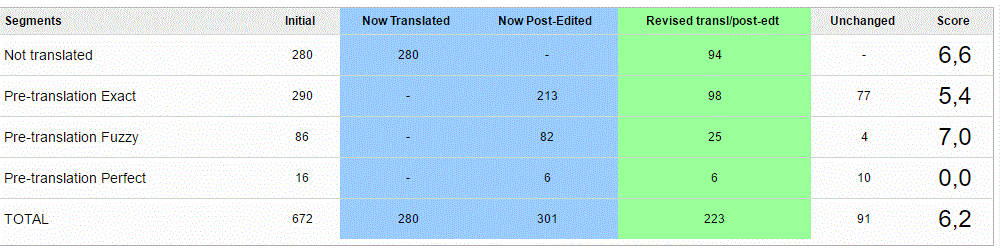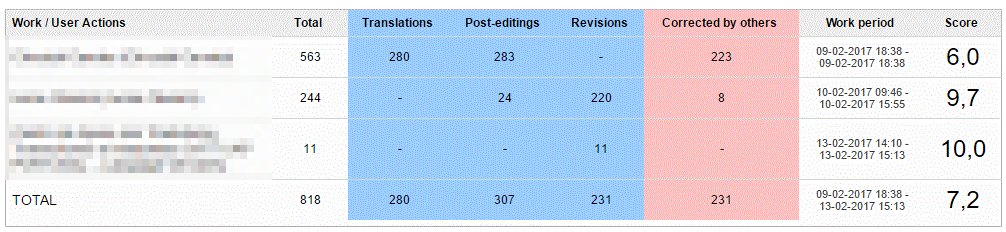Problem:

user does not know how calculations in the Translation Report have been performed.

1. First tableHow is the score calculated? In the screenshot above, Not Translated has a score of 6,6. How did Wordbee get that score? And how was the TOTAL score of 6,2 calculated?

Second tableis the translator's score affected by the score of Not Translated segments in the first table? As you can see the scores are very similar, i.e., 6,6 and 6,0.

The score is calculated based on the good translation (which is done) which hasn't been modified during revision or post editing.
In this example, calculations are as follows:
Translation score = [(280 now translated - 94 revised) /  280]*10 =  6,6
Pre-translation exact score = [(213 - 98) / 213]*10 = 5,4
....
The total ={ [(280 + 301) - 223 ] / (280+301)}*10 = 6.2For the second screenshot, the score is calculated based on the good work that is not corrected by others
First row supplier score = {[(280 + 283) - 223] / (280 + 283)}*10 = 6.0